# DAV Class 7 Science Chapter 3 Notes – Chemical Substances and Processes

These DAV Class 7 Science Notes and DAV Class 7 Science Chapter 3 Notes – Chemical Substances and Processes act as excellent revision resources, particularly in preparation for board exams.

## Chemical Substances and Processes Class 7 DAV Notes

→ Pure substance: Materials which are made of same kind of particles (atoms or molecules), are called pure substances. Example; iron, sodium chloride.

→ Element: An element is a pure substance which is made up of atoms of the same kind. Example; iron, copper, etc.

→ Compound: A compound is a pure substance which is made up of molecules of the same kind. A molecule can be made up of two or more elements. Example; sodium chloride, copper sulphate, etc.

→ Chemical Symbols: Chemical substances are usually represented by symbols. Nowadays, elements are represented by the letters of English alphabet. For example; the chemical symbol of iron is Fe, the chemical symbol of copper is Cu.

→ Chemical Formula: A substance can be shown by a chemical formula which shows the composition of that substance. For example; the chemical formula of water is H2O. Flere, H is the symbol of hydrogen and O is the symbol of oxygen. Similarly, the chemical formula of carbon dioxide is CO2. Flere, C is the symbol of carbon and O is the symbol of oxygen.→ Writing the chemical formula:

Symbols of positive and negative ions are written and their charge is written on the right corner of the symbol.
If there is any common factor, it is removed from the charges on the two ions.
Charges of two ions are criss-crossed and then written at the bottom right of the symbols of the two ions.

Some examples are given below: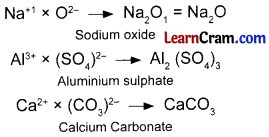→ Chemical Changes: When a new substance is formed during a change, the change is called a chemical change or chemical reaction.
→ Chemical Equation: Chemical reaction can be represented by using chemical symbols and formulae. Such a representation of a chemical reaction is called chemical equation.
In a chemical equation, the reactants are written on the left hand side of the equation and products are written on the right hand side of the equation. An arrow is put between them.
Reactants → Products
Physical states of reactants and products are shown by using ‘s’, T and ‘g’ respectively for solid, liquid and gas. For aqueous solution, ‘aq’ is written in bracket.
Example: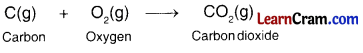→ Balancing of chemical equation:
When number of each element is same on both sides of the equation, the equation is called a balanced equation. For balancing a chemical equation, follow these steps:

→ Do not change the formula of a chemical substance in the reaction.
→ Write a suitable coefficient (number) with the formula of that substance; in order to balance the equation. Let us take following example: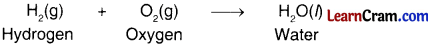In this equation, number of hydrogen atoms on both sides is same, but number of oxygen atom is not same.
H1(g) + O1(g) → 2H1O(l)
To balance the number of oxygen atoms, coefficient 2 is written with the formula of water on the right hand side of the equation.
2H1(g) + O1(g) → 2H2O(l)
Now, to balance the number of hydrogen atoms, coefficient 2 is written with the formula of hydrogen on the left hand side of the equation. Now, the equation is balanced.→ Types of Chemical Reaction:
1. Combination Reaction: When two or more substances combine to form a new substance, the reaction is called combination reaction. Examples:
Hydrogen and oxygen combine to form water. This can be shown by the following equation.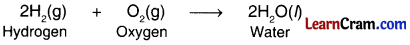Nitrogen and hydrogen combine to form ammonia. This can be shown by the following equation.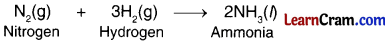2. Decomposition Reaction: When a substance gives two or more substances, the reaction is called decomposition reaction. Example:
Water molecule gets broken into hydrogen and oxygen when electric current is passed through it. This can be shown by the following equation.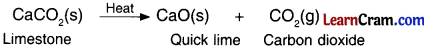When calcium carbonate is heated strongly, it decomposes into quick lime and carbon dioxide gas. This can be shown by the following equation.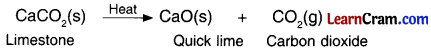3. Displacement Reaction: When one element displaces another element in a compound, the reaction is called displacement reaction. Example:
When iron nail is put into copper sulphate solution, iron displaces copper and forms iron sulphate. As a result, the blue colour of copper sulphate solution fades and the solution changes into green colour. This can be shown by following equation.4. Neutralization Reaction: When an acid and a base react with each other, salt and water are formed. This reaction is called neutralization reaction. Example:
Acid + Base → Salt + Water

When sodium hydroxide (a base) reacts with hydrochloric acid, sodium chloride (a salt) and water are formed. This can be shown by following equation.When magnesium hydroxide reacts with sulphuric acid, magnesium sulphate and water are formed. This can be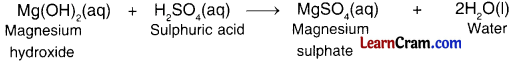→ Chemical reaction: The change in which a new substance is formed is called chemical reaction.

→ Pure substance: A substance which is composed of the same element or same molecule.

→ Element: A material which is composed of the same substance.→ Molecule: A material which is composed of one or more than one element is called molecule.

→ Chemical equation: Symbolic representation of a chemical reaction by using symbols is called chemical equation.

→ Combination reaction: A reaction in which two or more substances combine to form a new substance.

→ Decomposition reaction: A reaction in which a given substance decomposes into two or more substance.

→ Displacement reaction: A reaction in which an element displaces another element from a compound.

→ Neutralization reaction: Reaction between an acid and a base; to produce salt and water.

→ Matter: Anything which occupies space and has mass is called matter.

→ Solid: A matter which has definite shape and volume.

→ Liquid: A matter which has indefinite shape by definite volume.

→ Gas: A matter which has indefinite shape and volume.